• 矩阵特征值与特征向量 相似矩阵 实对称矩阵的对角化 向量的内积 实对称矩阵的对角化 矩阵特征值与特征向量矩阵AA是nn阶方阵，如果存在数λ\lambda和非零向量xx，使得Ax=λx，(1)Ax=\lambda x ，\...


矩阵的特征值与特征向量
相似矩阵
实对称矩阵的对角化
向量的内积
实对称矩阵的对角化

矩阵的特征值与特征向量

设矩阵A$A$$A$是n$n$$n$阶方阵，如果存在数λ$\lambda$$\lambda$和非零向量x$x$$x$，使得Ax=λx，(1)$\begin{array}{}\text{(1)}& Ax=\lambda x，\end{array}$Ax=\lambda x ，\tag{1}则称λ$\lambda$$\lambda$为矩阵A$A$$A$的特征值，称x$x$$x$为矩阵A$A$$A$对应特征值λ$\lambda$$\lambda$的一个特征向量.式（1）可写成（A−λE）x=0，(2)$\begin{array}{}\text{(2)}& （A-\lambda E）x=0，\end{array}$（A-\lambda E）x=0，\tag{2}上式说明齐次线性方程组（2）有非零解x$x$$x$，由齐次线性方程组有非零解的充分必要条件，得|A−λE|=0$|A-\lambda E|=0$$|A-\lambda E|=0$，即|A−λE|=∣∣∣∣∣∣∣a11−λa21...an1a12a22−λ...an2.........a1na2n...ann−λ∣∣∣∣∣∣∣=0.(3)$\begin{array}{}\text{(3)}& |A-\lambda E|=|\begin{array}{cccc}{a}_{11}-\lambda & {a}_{12}& ...& {a}_{1n}\\ {a}_{21}& a22-\lambda & ...& {a}_{2n}\\ ...& ...& & ...\\ {a}_{n1}& {a}_{n2}& ...& {a}_{nn}-\lambda \end{array}|=0.\end{array}$|A-\lambda E|=\left| \begin{matrix} a_{11}-\lambda & a_{12} & ... & a_{1n} \\ a_{21} & a{22}-\lambda & ... & a_{2n}\\ ... &...&&...\\ a_{n1} & a_{n2} & ... & a_{nn}-\lambda \end{matrix} \right| = 0 .\tag{3}记f(λ)=|A−λE|，f(λ)$f\left(\lambda \right)=|A-\lambda E|，f\left(\lambda \right)$$f(\lambda)=|A-\lambda E|，f(\lambda)$是关于λ$\lambda$$\lambda$的一个n$n$$n$次多项式，称f(λ)$f\left(\lambda \right)$$f(\lambda)$为矩阵A$A$$A$的特征多项式.特征多项式f(λ)$f\left(\lambda \right)$$f(\lambda)$的根就是矩阵A$A$$A$的特征值.
在复数范围内，n$n$$n$次多项式有n$n$$n$个根（重根按重数计算）.设λ1,λ2,...,λn${\lambda }_{1},{\lambda }_{2},...,{\lambda }_{n}$$\lambda_1,\lambda_2,...,\lambda_n$是n$n$$n$阶方阵A$A$$A$的n$n$$n$个特征值（重根按重数计算），利用根与系数之间的关系，有
λ1+λ2+...+λn=a11+a22+...+ann${\lambda }_{1}+{\lambda }_{2}+...+{\lambda }_{n}={a}_{11}+{a}_{22}+...+ann$$\lambda_1+\lambda_2+...+\lambda_n=a_{11}+a_{22}+...+a{nn}$；
λ1⋅λ2⋅...⋅λn=|A|${\lambda }_{1}\cdot {\lambda }_{2}\cdot ...\cdot {\lambda }_{n}=|A|$$\lambda_1 \cdot \lambda_2 \cdot ... \cdot \lambda_n=|A|$
设λ0${\lambda }_{0}$$\lambda_0$是n$n$$n$阶方阵A$A$$A$的一个特征值，则|A−λ0E|=0$|A-{\lambda }_{0}E|=0$$|A-\lambda_0E|=0$，从而齐次线性方程组(A−λ0E)x=0$\left(A-{\lambda }_{0}E\right)x=0$$(A-\lambda_0E)x=0$有非零解，其非零解就是矩阵A$A$$A$对应特征值λ0${\lambda }_{0}$$\lambda_0$的特征向量，所有非零解即为矩阵A$A$$A$对应于特征值λ0${\lambda }_{0}$$\lambda_0$的全部特征向量
n$n$$n$阶矩阵A$A$$A$与它的转置矩阵AT${A}^{T}$$A^T$具有相同的特征多项式，从而具有相同的特征值.事实上，|AT−λE|=|(A−λE)T|=|A−λE|.$|{A}^{T}-\lambda E|=|\left(A-\lambda E{\right)}^{T}|=|A-\lambda E|.$|A^T-\lambda E|=|(A-\lambda E)^T|=|A-\lambda E|.
设λ$\lambda$$\lambda$是n$n$$n$阶矩阵A$A$$A$的特征值，则
λ2${\lambda }^{2}$$\lambda^2$是A2${A}^{2}$$A^2$的特征值；
当A$A$$A$可逆时，1λ$\frac{1}{\lambda }$$\frac{1}{\lambda}$是A−1${A}^{-1}$$A^{-1}$的特征值
设λ1,λ2,...,λm${\lambda }_{1},{\lambda }_{2},...,{\lambda }_{m}$$\lambda_1,\lambda_2,...,\lambda_m$是n$n$$n$阶矩阵A$A$$A$的m$m$$m$个互不相等的特征值，其对应的特征向量分别为p1,p2,...,pm${p}_{1},{p}_{2},...,{p}_{m}$$p_1,p_2,...,p_m$，则p1,p2,...,pm${p}_{1},{p}_{2},...,{p}_{m}$$p_1,p_2,...,p_m$线性无关

相似矩阵

设矩阵A,B$A,B$$A,B$都是n$n$$n$阶方阵，如果存在可逆矩阵P$P$$P$，使P−1AP=B${P}^{-1}AP=B$$P^{-1}AP=B$，则称矩阵A，B$A，B$$A，B$相似.称变换P−1AP${P}^{-1}AP$$P^{-1}AP$为相似变换
相似矩阵具有相同的特征多项式，从而具有相同的特征值
如果矩阵A$A$$A$与对角矩阵Λ=⎛⎝⎜⎜⎜⎜λ1λ2...λn⎞⎠⎟⎟⎟⎟$\mathrm{\Lambda }=\left(\begin{array}{c}{\lambda }_{1}\\ & {\lambda }_{2}\\ & & ...\\ & & & {\lambda }_{n}\end{array}\right)$\Lambda=\left( \begin{matrix} \lambda_1 \\ &\lambda_2\\&&...\\&&&\lambda_n \end{matrix} \right)相似，则λ1,λ2...,λn${\lambda }_{1},{\lambda }_{2}...,{\lambda }_{n}$$\lambda_1,\lambda_2...,\lambda_n$就是矩阵A$A$$A$的特征值
如果n$n$$n$阶矩阵A$A$$A$与对角矩阵Λ$\mathrm{\Lambda }$$\Lambda$相似，则称矩阵A$A$$A$可相似对角化（简称为可对角化）
n$n$$n$阶矩阵A$A$$A$与对角矩阵Λ=⎛⎝⎜⎜⎜⎜λ1λ2...λn⎞⎠⎟⎟⎟⎟$\mathrm{\Lambda }=\left(\begin{array}{c}{\lambda }_{1}\\ & {\lambda }_{2}\\ & & ...\\ & & & {\lambda }_{n}\end{array}\right)$$\Lambda=\left( \begin{matrix} \lambda_1 \\ &\lambda_2\\&&...\\&&&\lambda_n \end{matrix} \right)$相似的充分必要条件是矩阵A$A$$A$有n$n$$n$个线性无关的特征向量
n$n$$n$阶矩阵A$A$$A$与对角矩阵Λ$\mathrm{\Lambda }$$\Lambda$相似的充分必要条件是矩阵A$A$$A$的特征值的重数等于其对应的线性无关的特征向量的个数

实对称矩阵的对角化

向量的内积

设有n$n$$n$维向量x=⎛⎝⎜⎜⎜⎜⎜⎜⎜x1x2...xn⎞⎠⎟⎟⎟⎟⎟⎟⎟，y=⎛⎝⎜⎜⎜⎜⎜⎜⎜y1y2...yn⎞⎠⎟⎟⎟⎟⎟⎟⎟，$x=\left(\begin{array}{c}{x}_{1}\\ {x}_{2}\\ .\\ .\\ .\\ {x}_{n}\end{array}\right)，y=\left(\begin{array}{c}{y}_{1}\\ {y}_{2}\\ .\\ .\\ .\\ {y}_{n}\end{array}\right)，$x=\left( \begin{matrix} x_1 \\ x_2 \\.\\.\\.\\x_n \end{matrix} \right)，y=\left( \begin{matrix} y_1 \\ y_2 \\.\\.\\.\\y_n \end{matrix} \right)，称x1y1+x2y2+...+xnyn${x}_{1}{y}_{1}+{x}_{2}{y}_{2}+...+{x}_{n}{y}_{n}$$x_1y_1+x_2y_2+...+x_ny_n$为向量x$x$$x$与y$y$$y$的内积，记为[x,y]$\left[x,y\right]$$[x,y]$，即[x,y]=xTy=x1y1+x2y2+...+xnyn$\left[x,y\right]={x}^{T}y={x}_{1}{y}_{1}+{x}_{2}{y}_{2}+...+{x}_{n}{y}_{n}$[x,y]=x^Ty=x_1y_1+x_2y_2+...+x_ny_n
内积是两个向量之间的一种运算，其结果是一个实数.内积满足以下性质：
[x,y]=[y,x]；$\left[x,y\right]=\left[y,x\right]；$$[x,y]=[y,x]；$
[λx,y]=λ[x,y]；$\left[\lambda x,y\right]=\lambda \left[x,y\right]；$$[\lambda x,y]=\lambda[x,y]；$
[x+y,z]=[x,z]+[y,z]；$\left[x+y,z\right]=\left[x,z\right]+\left[y,z\right]；$$[x+y,z]=[x,z]+[y,z]；$
[x,x]≥0$\left[x,x\right]\ge 0$$[x,x]\ge 0$，当且仅当x=0$x=0$$x=0$时，[x,x]=0.$\left[x,x\right]=0.$$[x,x]=0.$
其中，x,y,z$x,y,z$$x,y,z$都为n$n$$n$维向量，λ∈R.$\lambda \in R.$$\lambda \in R.$
设x=⎛⎝⎜⎜⎜⎜⎜⎜⎜x1x2...xn⎞⎠⎟⎟⎟⎟⎟⎟⎟∈Rn$x=\left(\begin{array}{c}{x}_{1}\\ {x}_{2}\\ .\\ .\\ .\\ {x}_{n}\end{array}\right)\in {R}^{n}$$x=\left( \begin{matrix} x_1\\x_2\\.\\.\\.\\x_n \end{matrix} \right) \in R^n$，称[x,x]‾‾‾‾‾√=x21+x22+...+x2n‾‾‾‾‾‾‾‾‾‾‾‾‾‾‾√$\sqrt{\left[x,x\right]}=\sqrt{{x}_{1}^{2}+{x}_{2}^{2}+...+{x}_{n}^{2}}$$\sqrt{[x,x]}=\sqrt{x_1^2+x_2^2+...+x_n^2}$为向量x$x$$x$的长度（或范数），记为||x||$||x||$$||x||$，即||x||=[x,x]‾‾‾‾‾√=x21+x22+...+x2n‾‾‾‾‾‾‾‾‾‾‾‾‾‾‾√$||x||=\sqrt{\left[x,x\right]}=\sqrt{{x}_{1}^{2}+{x}_{2}^{2}+...+{x}_{n}^{2}}$$||x||=\sqrt{[x,x]}=\sqrt{x_1^2+x_2^2+...+x_n^2}$
向量的范数具有以下性质：
非负性：||x||≥0$||x||\ge 0$$||x||\ge 0$，当且仅当x=0$x=0$$x=0$时，||x||=0$||x||=0$$||x||=0$；
齐次性：||λx||=|λ|⋅||x||$||\lambda x||=|\lambda |\cdot ||x||$$||\lambda x||=|\lambda|\cdot||x||$；
三角不等式：||x+y||≤||x||+||y||$||x+y||\le ||x||+||y||$$||x+y||\le ||x||+||y||$；
对任意n$n$$n$维向量x,y$x,y$$x,y$，有|[x,y]|≤||x||⋅||y||$|\left[x,y\right]|\le ||x||\cdot ||y||$$|[x,y]|\le ||x||\cdot ||y||$.
若令x=⎛⎝⎜⎜⎜⎜⎜⎜⎜x1x2...xn⎞⎠⎟⎟⎟⎟⎟⎟⎟，y=⎛⎝⎜⎜⎜⎜⎜⎜⎜y1y2...yn⎞⎠⎟⎟⎟⎟⎟⎟⎟，$x=\left(\begin{array}{c}{x}_{1}\\ {x}_{2}\\ .\\ .\\ .\\ {x}_{n}\end{array}\right)，y=\left(\begin{array}{c}{y}_{1}\\ {y}_{2}\\ .\\ .\\ .\\ {y}_{n}\end{array}\right)，$$x=\left( \begin{matrix} x_1 \\ x_2 \\.\\.\\.\\x_n \end{matrix} \right)，y=\left( \begin{matrix} y_1 \\ y_2 \\.\\.\\.\\y_n \end{matrix} \right)，$则上述性质（4）可表示为：|∑i=1|nxiyi≤∑i=1nx2i‾‾‾‾‾‾⎷⋅∑i=1ny2i‾‾‾‾‾‾⎷.$|\sum _{i=1}{|}^{n}{x}_{i}{y}_{i}\le \sqrt{\sum _{i=1}^{n}{x}_{i}^{2}}\cdot \sqrt{\sum _{i=1}^{n}{y}_{i}^{2}}.$|\sum_{i=1}|^{n}x_iy_i\le \sqrt{\sum_{i=1}^{n}x_i^2}\cdot \sqrt{\sum_{i=1}^{n}y_i^2}.上述不等式称为施瓦茨不等式
当||x||=1$||x||=1$$||x||=1$时，称x$x$$x$为单位向量，
当x≠0,y≠0$x\ne 0,y\ne 0$$x\not= 0,y\not= 0$时，由施瓦茨不等式，有|[x,y]||x||⋅||y|||≤1，$|\frac{\left[x,y\right]}{||x||\cdot ||y||}|\le 1，$|\frac{[x,y]}{||x||\cdot||y||}|\le 1，称θ$\theta$$\theta$为n$n$$n$维向量x$x$$x$与y$y$$y$的夹角
当[x,y]=0$\left[x,y\right]=0$$[x,y]=0$时，称向量x$x$$x$与y$y$$y$正交.显然，若x=0$x=0$$x=0$，则x$x$$x$与任何向量都正交.
若n$n$$n$维向量α1,α2,...,αr${\alpha }_{1},{\alpha }_{2},...,{\alpha }_{r}$$\alpha_1,\alpha_2,...,\alpha_r$是一组两两正交的非零向量，则α1,α2,...,αr${\alpha }_{1},{\alpha }_{2},...,{\alpha }_{r}$$\alpha_1,\alpha_2,...,\alpha_r$线性无关
设n$n$$n$维向量e1,e2,...,er${e}_{1},{e}_{2},...,{e}_{r}$$e_1,e_2,...,e_r$是向量空间V(V⊂Rn)$V\left(V\subset {R}^{n}\right)$$V(V\subset R^n)$的一个基，如果e1,e2,...,er${e}_{1},{e}_{2},...,{e}_{r}$$e_1,e_2,...,e_r$两两正交，且为单位向量，则称e1,e2,...,er${e}_{1},{e}_{2},...,{e}_{r}$$e_1,e_2,...,e_r$为向量空间V$V$$V$的一个规范正交基（或标准正交基）
设α1,α2,...,αr${\alpha }_{1},{\alpha }_{2},...,{\alpha }_{r}$$\alpha_1,\alpha_2,...,\alpha_r$是向量空间V$V$$V$的一个基，为了得到与α1,α2,...,αr${\alpha }_{1},{\alpha }_{2},...,{\alpha }_{r}$$\alpha_1,\alpha_2,...,\alpha_r$等价的一个规范正交基e1,e2,...,er${e}_{1},{e}_{2},...,{e}_{r}$$e_1,e_2,...,e_r$.这一过程，称为把基α1,α2,...,αr${\alpha }_{1},{\alpha }_{2},...,{\alpha }_{r}$\alpha_1,\alpha_2,...,\alpha_r规范正交化，可按如下两个步骤进行：
正交化：令
β1=α1${\beta }_{1}={\alpha }_{1}$$\beta_1=\alpha_1$;
β2=α−[β1,α2][β1,β1]β1${\beta }_{2}=\alpha -\frac{\left[{\beta }_{1},{\alpha }_{2}\right]}{\left[{\beta }_{1},{\beta }_{1}\right]}{\beta }_{1}$$\beta_2=\alpha-\frac{[\beta_1,\alpha_2]}{[\beta_1,\beta_1]}\beta_1$；
……
βr=αr−[β1,αr][β1,β1]β1−[β2,αr][β2,β2]β2−...−−[βr−1,αr][βr−1,βr−1]βr−1.${\beta }_{r}={\alpha }_{r}-\frac{\left[{\beta }_{1},{\alpha }_{r}\right]}{\left[{\beta }_{1},{\beta }_{1}\right]}{\beta }_{1}-\frac{\left[{\beta }_{2},{\alpha }_{r}\right]}{\left[{\beta }_{2},{\beta }_{2}\right]}{\beta }_{2}-...--\frac{\left[{\beta }_{r-1},{\alpha }_{r}\right]}{\left[{\beta }_{r-1},{\beta }_{r-1}\right]}{\beta }_{r-1}.$$\beta_r=\alpha_r-\frac{[\beta_1,\alpha_r]}{[\beta_1,\beta_1]}\beta_1-\frac{[\beta_2,\alpha_r]}{[\beta_2,\beta_2]}\beta_2-...--\frac{[\beta_{r-1},\alpha_r]}{[\beta_{r-1},\beta_{r-1}]}\beta_{r-1}.$容易验证β1,β2,...,βr${\beta }_{1},{\beta }_{2},...,{\beta }_{r}$$\beta_1,\beta_2,...,\beta_r$两两正交，且β1,β2,...,βr${\beta }_{1},{\beta }_{2},...,{\beta }_{r}$$\beta_1,\beta_2,...,\beta_r$与α1,α2,...,αr${\alpha }_{1},{\alpha }_{2},...,{\alpha }_{r}$$\alpha_1,\alpha_2,...,\alpha_r$等价.上述过程也称为施密特正交化
单位化：令e1=1||β1||β1，e2=1||β2||β2，...，er=1||βr||βr，${e}_{1}=\frac{1}{||{\beta }_{1}||}{\beta }_{1}，{e}_{2}=\frac{1}{||{\beta }_{2}||}{\beta }_{2}，...，{e}_{r}=\frac{1}{||{\beta }_{r}||}{\beta }_{r}，$e_1=\frac{1}{||\beta_1||}\beta_1，e_2=\frac{1}{||\beta_2||}\beta_2，...，e_r=\frac{1}{||\beta_r||}\beta_r，则e1,e2,...,er${e}_{1},{e}_{2},...,{e}_{r}$$e_1,e_2,...,e_r$是向量空间V$V$$V$的一个规范正交基
如果n$n$$n$阶矩阵A$A$$A$满足ATA=E，即A−1=AT，${A}^{T}A=E，即{A}^{-1}={A}^{T}，$A^TA=E，即A^{-1}=A^T，则称矩阵A$A$$A$为正交矩阵，简称正交阵
正交矩阵具有以下性质：
如果矩阵A$A$$A$是正交矩阵，则|A|=1$|A|=1$$|A|=1$或(−1)$\left(-1\right)$$(-1)$；
如果矩阵A，B$A，B$$A，B$都是正交矩阵，则AB$AB$$AB$也是正交矩阵
n$n$$n$阶矩阵A$A$$A$为正交矩阵的充分必要条件是A$A$$A$的列向量组是两两正交的单位向量组.

实对称矩阵的对角化

实对称矩阵的特征值一定是实数
设λ1，λ2${\lambda }_{1}，{\lambda }_{2}$$\lambda_1，\lambda_2$是实对称矩阵A$A$$A$的两个不相等的特征值，其对应的特征向量分别为p1,p2${p}_{1},{p}_{2}$$p_1,p_2$，则p1${p}_{1}$$p_1$与p2${p}_{2}$$p_2$正交
设A$A$$A$为n$n$$n$阶实对称矩阵，λ$\lambda$$\lambda$是A$A$$A$的特征方程的k$k$$k$重根，则矩阵A−λE$A-\lambda E$$A-\lambda E$的秩R(A−λE)=n−k$R\left(A-\lambda E\right)=n-k$$R(A-\lambda E)=n-k$，从而对应特征值λ$\lambda$$\lambda$恰有k$k$$k$个线性无关的特征向量
设A$A$$A$为n$n$$n$阶实对称矩阵，则必有正交矩阵P$P$$P$，使P−1AP=PTAP=Λ${P}^{-1}AP={P}^{T}AP=\mathrm{\Lambda }$$P^{-1}AP=P^TAP=\Lambda$.其中Λ$\mathrm{\Lambda }$$\Lambda$是以A$A$$A$的n$n$$n$个特征值为对角元素的对角矩阵.
将一个n$n$$n$阶实对称矩阵A$A$$A$对角化的步骤为：
求出A$A$$A$的全部互不相等的特征值λ1,λ2,...,λs${\lambda }_{1},{\lambda }_{2},...,{\lambda }_{s}$$\lambda_1,\lambda_2,...,\lambda_s$，它们的重数分别k1,k2,...,ks(k1+k2+...+ks=n)${k}_{1},{k}_{2},...,{k}_{s}\left({k}_{1}+{k}_{2}+...+{k}_{s}=n\right)$$k_1,k_2,...,k_s(k_1+k_2+...+k_s=n)$；
对每个ki${k}_{i}$$k_i$重特征值λi${\lambda }_{i}$$\lambda_i$，由(A−λiE)x=0$\left(A-{\lambda }_{i}E\right)x=0$$(A-\lambda_iE)x=0$求出基础解系，得ki${k}_{i}$$k_i$个线性无关的特征向量，把它们正交化、单位化，便得n$n$$n$个两两正交的单位特征向量p1,p2,...,pn${p}_{1},{p}_{2},...,{p}_{n}$$p_1,p_2,...,p_n$；
令P=(p1,p2,...,pn)$P=\left({p}_{1},{p}_{2},...,{p}_{n}\right)$$P=(p_1,p_2,...,p_n)$，便有P−1AP=PTAP=Λ${P}^{-1}AP={P}^{T}AP=\mathrm{\Lambda }$$P^{-1}AP=P^TAP=\Lambda$

展开全文线性代数
• 最近项目中有一个模块需要求矩阵的最大特征值和特征值对应的特征向量，无奈，又重新将以前学习的这方面的知识重新温习了一遍，感觉还是当时学的不够深，所以谢谢感悟，顺便对知识点进行一个总结。 首先特征值和特征...
最近项目中有一个模块需要求矩阵的最大特征值和特征值对应的特征向量，无奈，又重新将以前学习的这方面的知识重新温习了一遍，感觉还是当时学的不够深，所以谢谢感悟，顺便对知识点进行一个总结。

首先特征值和特征向量的求解根据项目的需求或者是矩阵的具体形式，主要可以分成如下三种形式：

自己只需要获得矩阵的最大特征值和特征值所对应的特征向量
需要求取矩阵的所有特征值
需要求取特征值和特征向量的矩阵为实对称矩阵，则可以通过另一种方法进行求解
现在我们来分析这三种形式特征值和特征向量的求取：

1.如果自己仅仅要求最大特征值的话肯定采用形式1的算法，该算法的优点是时间复杂度较低，计算量相对较小，该方法不但能够求取特征值和特征向量，而且只要特征值不全为0，该方法都能获得想要的结果。

2.如果需要获得一个矩阵的所有特征值，则通过形式2可以很好的解决该问题，但是该方法的缺点是仅仅能够获得特征值，获得特征值之后利用其它方法进行求解，这样做自然而然计算量就大了起来。

3.如果矩阵为实对称矩阵，那么可以通过形式3对其进行特征值和特征向量的求取，该方法相对于形式2的好处就是能够一次性将特征值和特征向量求取出来，缺点就是矩阵必须是实对称矩阵，至于算法复杂度方面我没有进行测试，不过猜测一下应该形式3复杂度相对来说要低一点（不然这种算法毫无有点怎么可能存活下来）。

下面对上面三种形式采用的算法进行说明：

乘幂法

乘幂法主要针对求取矩阵的最大特征值和特征向量，原理就是迭代求极限，推导过程如下：

首先我们不妨假设矩阵A的n个特征值的关系如下：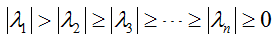（1）

且矩阵有相应的n个线性无关的特征向量x1,x2,x3.....xn，如果学过矩阵论就会知道，上述n个线性无关特征向量构成了n为线性空间的一组基，通俗的讲就是任意一个n维的一个向量都可以用上面n个向量进行表示，例如n维向量z0表示形式如下：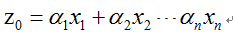（2）

在矩阵两边同时乘以矩阵A,则有：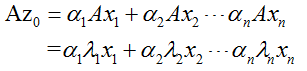（3）

当矩阵两边连乘n个矩阵A，则有：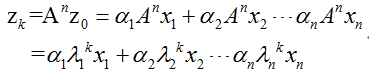（4）

将上式进行变换后可得：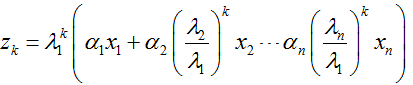（5）

由假设可知，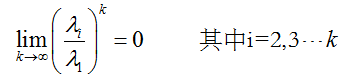，所以可以得到：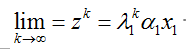（6）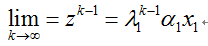（7）

由式（6）（7）联立可得矩阵最大特征值，结果如下：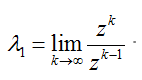（8）

已知特征值由式（7）可得相应的特征向量：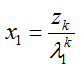（9）

由乘幂法的迭代过程容易看出，如果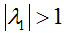或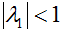，那么迭代向量zk的各个非零的分量将随着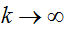而趋于无穷（或趋于零），这样在计算机上实现时就可能上溢(或下溢). 为了克服这个缺点，需将每步迭代向量进行规范化，过程如下：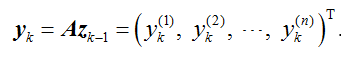（10）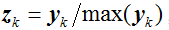（11）

定理

设A是n阶实矩阵，取初始向量z0，通常取z0={1,1,.....,1}，其迭代过程是：对k=1,2......，有: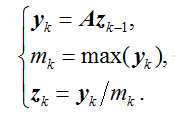(12)

通过迭代，有：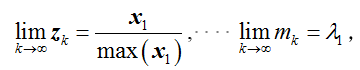（13）

证明过程如下：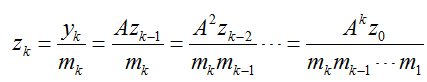（14）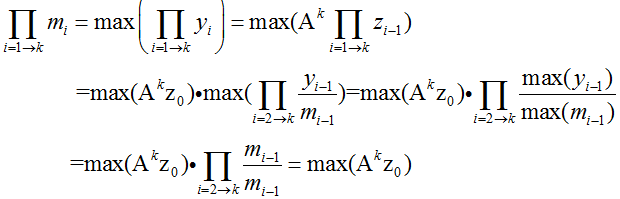（15）

由式（14）和（15）联立可得：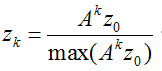（16）

由式（4）可知：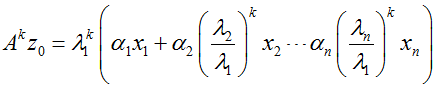（17）

则有：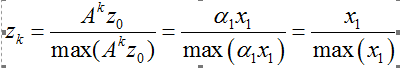（18）

定理第一个式子得证，下面证明第二个式子：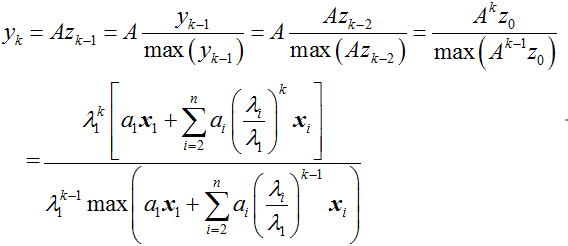（19）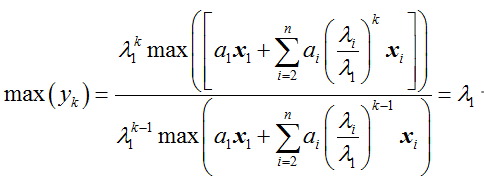（20）

由此定理得证。

由定理可知乘幂算法步骤如下：

（1）首先寻找任意向量z0,一般情况去z0={1,1,.....1}。

（2）由z0计算得到y1,而后寻找y1向量中的最大值m1。

（3）由y1和m1得到z1,判断迭代次数是否达到，如果达到则跳到步骤4，然否则跳到步骤2循环。

（4）此时获得的mk即矩阵A的特征值，zk即为相应的特征向量，算法结束。

注：这里迭代结束判断可以是迭代次数，也可以是特征值mi前后两次的误差，我写程序的时候采用的是误差判断。

至于具体的程序，目前还未整理好，注释啥的都没写，所以暂时先不放上，等把注释写好了再贴上来。

QR算法

QR算法是针对解决形式2的算法，要看懂该算法需要一点矩阵论里面的知识，我尽量讲的通俗一点，如果还看不懂请翻翻矩阵论第四章矩阵分解的知识点。

1.QR分解

任意一个矩阵A可以分解成如下两个矩阵表达的形式：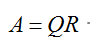（21）

其中矩阵Q为正交矩阵，矩阵R为上三角矩阵，至于QR分解到底是怎么回事，矩阵Q和矩阵R是怎么得到的，你们还是看矩阵论吧，如果我把这些都介绍了，感觉这篇文章要写崩，或者你可以先认可我是正确的，然后往下看。

首先我们有A1=A=QR，则令A2=RQ,则有：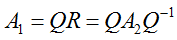（22）

由式（22）可知，A1和A2相似，相似矩阵具有相同的特征值，说明A1和A2的特征值相同，我们就可以通过求取A2的特征值来间接求取A1的特征值。

定理

设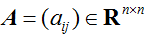, {Ak}是由QR算法产生的矩阵序列，其中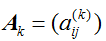,若满足如下条件：

（1）矩阵A的特征值满足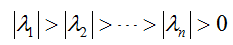（2）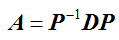，其中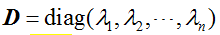，而且P有三角分解P=LU，(L是单位下三角矩阵，U是上三角矩阵)。

则有：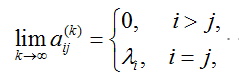（23）

上面的定理我就不证明了，因为我也不会，本人矩阵论学的也是二把刀，请见谅，但是看到上面的定理的两个条件是否能引起你的注意，首先是条件1，该条件严重限制了该方法的使用范围，矩阵的特征值不能相同且不能为0，条件2表明矩阵A能够对角化。

其大概原理就是如此，下面我们来说明QR算法的具体步骤：

（1）首先矩阵Ai进行QR分解，根据得到矩阵Qi和Ri

（2）根据矩阵Qi和Ri，得到Ai+1=Ri*Qi

（3）判断是否跳出循环条件，如果跳出循环条件则跳到步骤4，否则跳到步骤1进行循环

（4）由得到的矩阵Ai+1，该矩阵对角线上元素为矩阵A的特征向量。

Jacobi方法

Jacobi方法主要针对实对称矩阵，首先要对实对称矩阵的性质进行说明，实对称矩阵的特征向量都为正交向量，并且存在如下关系：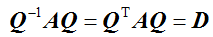上式中Q为正交矩阵，由此可见，Jacobi方法的实质和关键就是找一个正交矩阵Q，将矩阵A化为对角矩阵。

矩阵中存在两种线性变换，Givens变换和Householder变换，两种变换的共同点就是变换矩阵为正交阵。由此我们可以通过Givens变换或者Householder变换将矩阵A化为对角阵，而相应的变换矩阵的列向量即为特征向量，变换后的对角阵元素即为特征值。下面来介绍一波Givens变换：

Givens变换

设矩阵A是n阶实对称矩阵，称n阶矩阵,存在矩阵G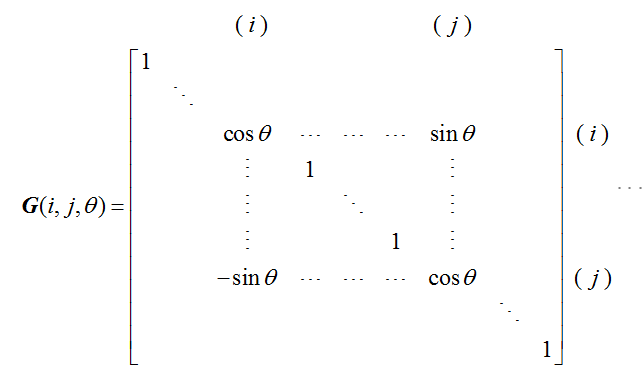上面矩阵G为Givens变换对应的变换矩阵（其本质是将向量逆时针旋转一定角度后的新向量），通过改变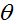左乘该矩阵可以将第i行第j列的元素变成0，通过(n-1)(n-2)/2次变换即可将n*n矩阵变换成对角阵，这就是Jacobi方法的原理。

注：左乘矩阵G只会改变原来矩阵第i行，第i列；第j行；第j列中的元素，所以通过下面式子可以很快利用原矩阵获得新矩阵的各个元素，计算式子如下：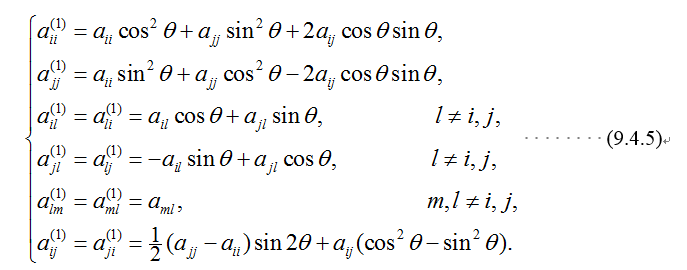不知道你们对上面的说的算法原理之后会不会产生一个疑问，如果我经过一次变换将一个元素变成0之后下一次变换后会不会该元素会不会又不为0了？？答案是肯定会的，但是为啥这样的算法还能用呢？？下面定理解决了这个疑问：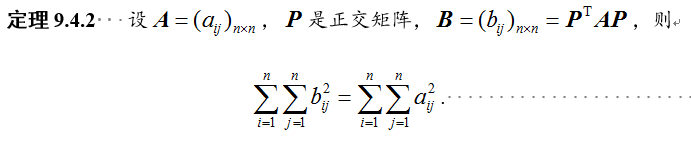上述定理可知，Givens变换后矩阵各个元素的总和是不变的，而可以证明，我们每次变换之后，非对角线上的元素的总和都变小了，相应的对角线上的元素都变大了，所以当非对角线上元素总和小于某个误差值时，我们可以认为对角线上的元素即为特征值，这就是上述定理的理论依据。

Jacobi算法步骤：

（1）找到矩阵Ai中非对角线上最大的元素，将利用Givens变换将该元素变换成0

（2）利用公式可以得到变换后的矩阵Ai+1。

（3）获得左乘矩阵Gi。

（4）计算变换后矩阵Ai+1的非对角线元素和，如果满足小于误差值的要求即跳到步骤5，否则跳到步骤1进行循环

（5）变换后矩阵Ak对角线元素即为特征值，变换矩阵Gk*Gk-1........G1连乘得到矩阵G,G的列向量即为特征向量。

至于代码，整理好再贴出来。


展开全文• 3.获取下三角阵 tril(A) 4.解线性方程组 Ax=b x=A\b或者x=inv(A)*b 5.计算A的特征值 lambda=eig(A) 其中，lambda是A的全部特征值构成的列向量 6.计算出A的全部特征值和对应的特征向量 [P, D]=eig(A) 其中，D是对角...
1.矩阵A的对角阵
diag(A)
2.获取上三角阵
triu(A)
3.获取下三角阵
tril(A)
4.解线性方程组
Ax=b
x=A\b或者x=inv(A)*b
5.计算A的特征值
lambda=eig(A)
其中，lambda是A的全部特征值构成的列向量
6.计算出A的全部特征值和对应的特征向量
[P, D]=eig(A)
其中，D是对角矩阵，保存A的全部特征值
P是满阵，P的列向量构成对应于D的特征向量组。


展开全文• 文章目录特征值和特征向量特征值的性质可对角化矩阵正定矩阵奇异矩阵正交矩阵QR分解(正交三角分解)施密特正交化(把线性无关组转换为正交组)SVD奇异值分解向量的导数标量对向量的导数标量对方阵的导数 特征值和特征...
文章目录特征值和特征向量特征值的性质可对角化矩阵正定矩阵奇异矩阵正交矩阵QR分解(正交三角分解)施密特正交化(把线性无关组转换为正交组)SVD奇异值分解向量的导数标量对向量的导数标量对方阵的导数
特征值和特征向量特征值的性质可对角化矩阵正定矩阵奇异矩阵正交矩阵QR分解(正交三角分解)施密特正交化(把线性无关组转换为正交组)SVD奇异值分解向量的导数标量对向量的导数标量对方阵的导数展开全文• 矩阵特征值，主要是用的QR分解，在我的有一次博客里，我已经详细地给出了计算的过程，大家有兴趣可以去看，经过几天的钻研，终于完成了整个的eig算法。下面我将把我的整个代码附上，有不懂的可以问我，欢迎...c
• 矩阵特征分解介绍及雅克比(Jacobi)方法实现特征值和特征向量的求解(C++/OpenCV/Eigen)
• 在计算出A的基础上，先利用Householder矩阵对矩阵A作相似变换，把A化为拟上三角矩阵A(n-1)，然后进行带双步位移的QR分解（其中Mk的QR分解可调用子程序），通过调用一元二次方程的根解二阶块矩阵的特征值，最后计算出...
• 设A是n阶矩阵，α是n维非零列向量，满足：Aα=λα，(1)Aα=λα，\tag1Aα=λα，(1)则称λ是A的一个特征值。非零列向量是A的属于λ的一个特征空间。 式（1）可以写成 (λEn−A)X=0(λE_n-A)X=0 (λEn​−A)X=0 它...线性代数
• 特征值与特征向量10.1.1. 标准特征值与特征向量问题10.1.2. 广义特征值与特征向量问题10.1.3. 部分特征值问题10.2. 矩阵对角化10.2.1. 预备知识10.2.2. 具体操作10.3. 若尔当(Jordan)标准形10.3.1. 若尔当标准形...matlab
• python 下三角矩阵A matrix can be seen in different ways and one of them is the upper triangular matrix part. Some problems in linear algebra are concerned with the upper triangular part of the matrix....python numpy 机器学习 深度学习 人工智能
• ## 矩阵和向量

千次阅读 2016-02-16 14:30:25
Math.Net Numerics对矩阵向量提供了丰富的类型.他们支持单精度,双精度类型和实数和复数类型. 像.Net的所有数据结构一样,他们都是基于0索引的.例如左上角的元素的索引是(0,0),在矩阵中第一个索引符指的是行,第二...
• #Python实现将Excel的数值型数据转成矩阵，并计算其特征值和特征向量的计算方法 import numpy as np import pandas as pd import xlrd from sklearn import preprocessing def excel_to_matrix(path): table = xlrd...python 数据分析 大数据
• 引入问题：给定一个对角线非零的上三角矩阵$$M$$，求$$M^k$$，满足$$M$$的阶\...一开始我想，既然是上三角矩阵，那么特征多项式一定不难求，那么是用CH定理+FFT多项式取模啥搞搞？ 然而我naive了。 这题我们可以把\(...
• 设A为矩阵 det（A）求矩阵的值 trace（A）求矩阵的迹 rank（A）求矩阵的秩 norm（A）求矩阵的范数 norm（A，1）求矩阵的1范数 norm（A，inf）求矩阵的无穷范数 ...
• ## 特征向量

千次阅读 2018-08-19 13:39:04
矩阵（这里特指方阵）是什么，从特征向量的角度来讲，矩阵其实是一个函数，一个作用在向量x的函数。 这些向量中我们感兴趣是那些向量呢，是那些经过变换前后方向一致的向量。这些向量是比较特殊的，对于多数的向量...
• 如果把矩阵看做运动的表征，那么 特征值就是运动的速度 特征向量就是运动的方向 Ref 如何理解矩阵特征值？ - 马同学的回答 - 知乎 矩阵特征值和特征向量的几何意义 - 百度文库 ...
• 对角矩阵(diagonal matrix)：只在主对角线上含有非零...我们用diag(v)表示一个对角元素由向量v中元素给定的对角方阵。对角矩阵受到关注的部分原因是对角矩阵的乘法计算很高效。计算乘法diag(v)x，我们只需要将x中...
• 如果：Ax=ax则a是矩阵A的特征值，x是矩阵A的属于特征值a的特征向量。x！=0即（aI-A）x=0有非0解即满足方程det(aI-A)=0的a都是A的特征值。特征值即是多项式det(aI-A)=0的根。（注：A为m*n的矩阵，齐次线性方程组Ax=0...c
• 如何使用mkl中使用dsyevd函数高效的求解大型实对称矩阵的所有特征向量特征matrix 存储 delete algorithm 语言
• 1、向量的创建 1）直接输入： 行向量：a=[1,2,3,4,5]列向量：a=[1;2;3;4;5] 　 2）用“:”生成向量 a=J:K 生成的行向量是a=[J,J+1,…,matlab 创建
• 二阶张量是矩阵向量的组合） 三阶张量是数据立体（矩阵的组合） 四阶张量（数据立体的组合） 等等。 1、纯量就是一个数值，可以看成是一个数值上的变化量。 2、向量是点到点的变化量，而点可以是一维空间上的...
• 第一节 向量的内积 一．数学概念 1. 内积：设有n维向量   令 ， 则称[x,y]为向量x与y的内积。 2. 范数：称 为向量x的范数(或长度)。 3. 单位向量：称 时的向量x为单位向量。 4. 当 ， 时，称   为向量x...线性代数
• 矩阵 1.方程组的几何解释 (1)行图像(row picture):1个行图像展示1个方程 (2)列图像(column picture):1个 (3)矩阵形式(matrix form) 2x-y=0 -x+2y=3 -- -- -- -- -- -- | 2 -1 | | x | = | 0 | | -1 2 | | y | | 3 |...线性代数 数学建模
• BLAS 2级函数(矩阵-向量操作) cblas_?gbmv(计算矩阵-向量乘积) cblas_sgbmv (const CBLAS_LAYOUT Layout, const CBLAS_TRANSPOSE trans, const MKL_INT m, const MKL_INT n, const MKL_INT kl, const MKL_INT ku, ...intel c++
• ## 特征值和特征向量

千次阅读 2014-07-12 22:34:26
特征值（eigenvalues）和特征向量（eigenvectors）都是对方阵而言的，给定矩阵A，它就像某个函数一样作用在向量x上，从而得到新向量Ax，我们感兴趣的是矩阵作用后那些新向量Ax与原向量x方向一致的向量，对多数向量而...
• 本文是Gilbert Strang的...对方阵的特征值和特征向量做讲解，矩阵特征值和特征向量会反映出矩阵的重要信息，后面的课将讲解特征值和特征向量的应用以及为什么需要特征值和特征向量特征向量特征值概念 Ax，矩阵共轭复数
• 定理 7.1 若A可逆，则存在正交矩阵Q和主对角为正数的上三角矩阵R，使得 A = Q R A = Q R A=QR 通过Schmidt正交化证明 定理 7.4 设线性变换 σ ∈ L ( V , V ) σ ∈ L ( V , V ) \sigma \in L(V,V) ， B ...
• 对角矩阵可以认为是矩阵中最简单的一种，值得一提的是：对角线上的元素可以为 0 或其他值，对角线上元素相等的对角矩阵称为数量矩阵；对角线上元素全为1的对角矩阵称为单位矩阵。对角矩阵的运算包括和、差运算、数乘...
• 首先对角线元素互不相同，意味着矩阵有n个不同的特征值，根据6.3.1知道，必定有n个线性无关的特征向量，故T可以对角化. 2, 然后取一个特征值tjj对应的特征向量是rj, (T- tjj * I)x=0 必定有非平凡解rj, 3.  A= T-......